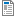National Curriculum

# Year 2 Maths Plans

We provide Hamilton Year 2 maths both as weekly plans (below) and as short blocks. We will eventually be phasing out the plans, as we believe our short blocks offer you all of the same advantages and more. Find out more about the advantages of Hamilton's short blocks.

In order to ensure that every part of the Year 2 content is covered before the statutory assessments in late May, there are nearly 13 weeks of teaching in the Autumn Term, 11 weeks in Spring and 4+ weeks before the SATs in Summer, plus some optional revision sessions. Each teacher will need to pace this teaching to suit their class and, crucially, their term dates. You may need to consider bringing some of the Spring Term teaching into Autumn. For more support in how to arrange this around different potential term structures structures, read more.

Supporting documents for set
• Week
• Title
1
+ Details
Sequences and place value

Mark 2-digit numbers on a beaded then landmarked line; order and compare numbers to 100; say a number between any given neighbouring pairs of multiples of ten; count on in tens from 1 and 2-digit numbers and back again; make a sensible estimate up to 100; show 2-digit numbers on a bead string and write the corresponding addition; partition 2-digit numbers into multiples of ten and one; mental addition and subtraction using place value/ partitioning.Autumn Week 1 Plan
•Autumn Week 1 Image Resource
•ITP Number Grid
2
+ Details
Number facts and counting

Mental addition and subtraction by partitioning all numbers to 10, then 20 into pairs; use the = sign to represent equality (e.g. 6 + 4 = 7 + 3); recognise the use of a symbol such as ■ to represent an unknown; count on in tens from any 1-digit number then any number, and back again; relate counting on/back in tens to finding 10 more/less. Includes bar model examples.Autumn Week 2 Plan
3
+ Details
Money and time

Coins: Recognise, find the total value of two, find totals up to 20p, find change from 20. Find all possibilities by making an ordered list. Read the time to the quarter of an hour on digital and analogue clocks, begin to identify time intervals. Mental addition using partitioning and adding near multiples. Mental subtraction using counting up. Includes bar model examples.

4
+ Details

Use pairs to ten to find the complement to the next multiple of ten; find change from 20p; add and subtract 10, 11 and 20 in the context of money. Mental addition using near multiples and partitioning. Mental subtraction using counting up. Includes bar model examples.

5
+ Details
Length, position and direction

Measure the length of objects using standard units (decimetre, centimetre and metre); identify left and right; recognise whole, half and quarter turns, both clockwise and anticlockwise; recognise that a right angle is a quarter turn.

6
+ Details
Sequences and fractions

Count in tens from any number: recognise multiples of 10; begin to use multiplication; count in twos: recognise odd and even numbers; find halves and quarters of shapes.

7
+ Details

Find doubles to double 20 and corresponding halves; add and subtract 10, 20, 11 and 21. Mental addition and subtraction using near multiples and partitioning. Includes bar model examples.

8
+ Details

Know pairs with a total of 20 and derive the subtraction facts; recognise the use of a symbol such as ■ to represent an unknown; add/subtract a single digit to/from a 2-digit number not crossing tens using number facts and pattern; add/subtract a single digit to/from a 2-digit number by bridging multiples of ten using knowledge of pairs to ten, place value/partitioning and counting up .

9
+ Details

Use pairs to ten to find the complement to the next multiple of ten; use place value to add and subtract; mental addition and subtraction of a single digit to/from a 2-digit number by bridging multiples of ten using partitioning, near multiples and place value; understand that addition can be done in any order, but not subtraction; sort calculations as to whether number facts or place value can be used to help identify the useful number fact.

10
+ Details
2D Shape and data

Recognise common 2D shapes, identify from pictures in different positions and orientations; draw, sort and describe 2D shapes, referring to their properties including symmetry and right angles (‘square’ corners).

11
+ Details